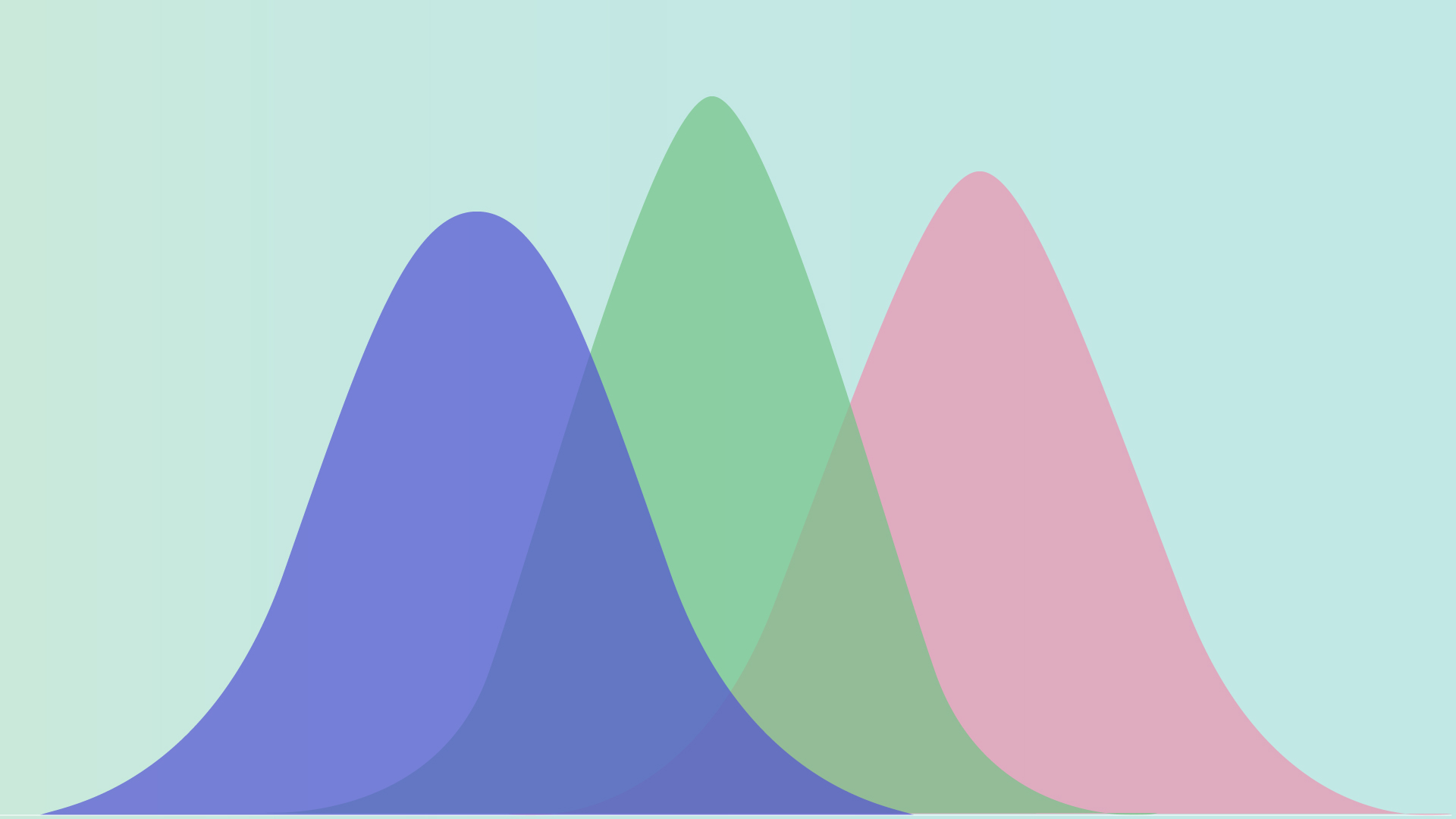## Course summary

• Type MOOC course
• Period Always open
• Learning Time Study freely
• Course approval method Automatic approval
• Certificate Not Issued
http://www.edwith.org/bayesiandeeplearning
Thumb up 545 Learner 5508### Instructor Introduction

•최성준

안녕하세요. 저는 현재 박사 과정 중에 있으며, 기계 학습과 로보틱스를 주로 연구하고 있습니다. 오랜 시간을 관악산에 거주하였으나 지금은 LA에서 리서치 인턴을 하고 있습니다. 대부분의 시간을 이런 저런 생각을 하거나 코딩을 하면서 보내고, 종종 논문을 읽고, 또 쓰기도 합니다. 최근엔 생활비를 벌기 위해서 강의도 가끔씩 합니다. 주로 연구하는 분야는 모방 학습과 강화 학습 분야이고, 관련 연구자들을 만나서 잡담하는 것을 좋아합니다. 연구 관련 연락은 언제든 환영이에요. :)

### Lecture plan

강의목록
1. CHAPTER 1. Elementary of mathematics
1. Introduction
1. Set theory
1. Measure theory
1. Probability
1. Random variable
1. Random process
1. Functional analysis
2. CHAPTER 2. Gaussian process
1. Introduction
1. Gaussian process
1. Weight space view
1. Function space view
1. Gaussian process latent variable model (GPLVM)
1. Gaussian process Application : 최성준님 연구 소개
3. CHAPTER 3. Bayesian deep neural network (1)
1. Introduction
1. Minimizing the Description Length
1. Ensemble Learning in Bayesian Neural Network
1. Practical variational inference
1. Bayes by backprop (BBB)
4. CHAPTER 4. Bayesian deep neural network (2)
1. Summary of Variational Inference
1. Dropout as a Bayesian Approximation
5. CHAPTER 5. Summary
1. Summary
6. CHAPTER 6. Uncertainties in Deep Learning
1. Introduction
1. Uncertainty in Deep Learning
1. Representing Inferential Uncertainty through Sampling
1. Bayesian Uncertainty Estimation
1. Predictive Uncertainty Estimation using Deep Ensembles
1. Uncertainties in Bayesian Deep Learning for Computer Vision
1. Uncertainty -Aware Reinforcement Learning
1. Safe Visual Navigation via Deep Learning
1. Uncertainty-Aware Learning using Mixture Density Networks